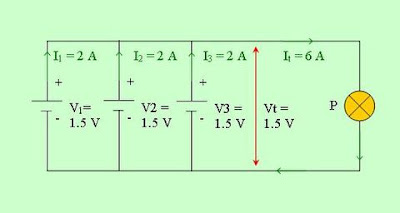# Calculation of parallel connection

In a closed circuit connected in parallel, the amount of voltage at any place in the circuit is the same. While the amount of current flow and the amount of resistance can vary. To determine the amount of voltage and the amount of electrical resistance in parallel circuit, can use the formula:

It = I1 + I2 + I3 + • • • • • • + In
1/Rt = 1/R1 + 1/R2 + 1/R3 + • • • • • • + 1/Rn
Vt = V1 = V2 = V3 = • • • • • • = Vn

Where:
- Vt = number of voltage
- Rt = total resistance
- It = number of flow
- V1, V2, V3 = voltage of each source
- R1, R2, R3 = resistance of each component
- I1, I2, I3 = flow through each channel
- Vn = voltage at the source n
- Rn = resistance at the n components
- In = current in the channel n

Sample question:
What is the amount of voltage and current generated in the installation of three parallel battery voltage and current are respectively 1.5 V and 2 A?
Completion:
It = I1 + I2 + I3 = 2 + 2 + 2 = 6 A
Vt = V1 = V2 = V3 = 1.5 V
The number of voltage generated is a fixed 1.5 V and current of 6 ANext→
←Prev

### Choose label

1.